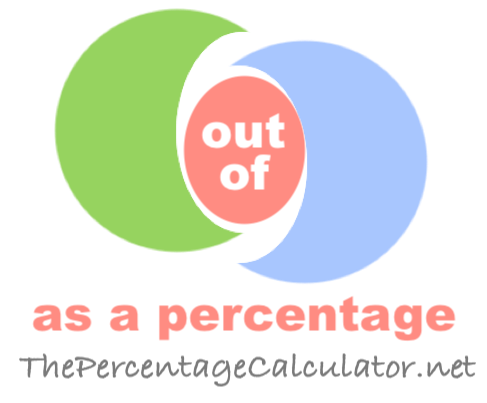Out of as a Percentage CalculatorThis Out of as a Percentage Calculator can calculate what a number is out of another number as a percentage.

In other words, you enter two numbers and we will tell you what portion the first number is in relation to the second number, expressed as a percentage.

out of

Here are some suggested calculations that you may find educational:

What is 3 out of 5 as a percentage?

What is 5 out of 20 as a percentage?

What is 10 out of 50 as a percentage?

What is 25 out of 75 as a percentage?